@coder-pig 2018-04-02T03:51:28.000000Z 字数 4894 阅读 2499

# 小猪的Python学习之旅 —— 14.爬虫实战：抓取豆瓣音乐Top 250数据存到Excel中

Pythonsplit("~")是目录，split("~")是路径，挺low的，## 1.编写抓数据脚本

• 1.数据能在Network选项卡找到，非JS动态加载，直接处理结果就好
• 2.点击第二页，选中XHR，没有东东，不是Ajax动态加载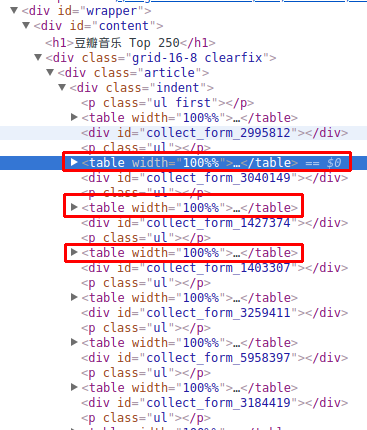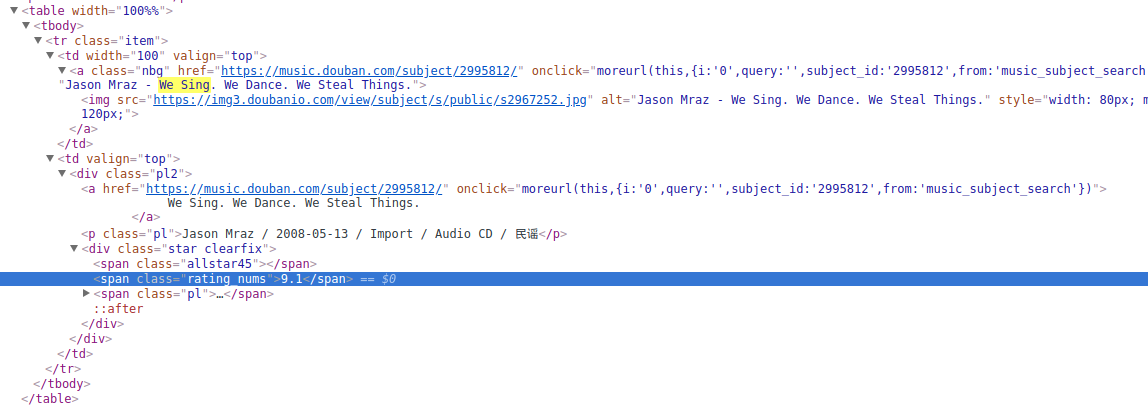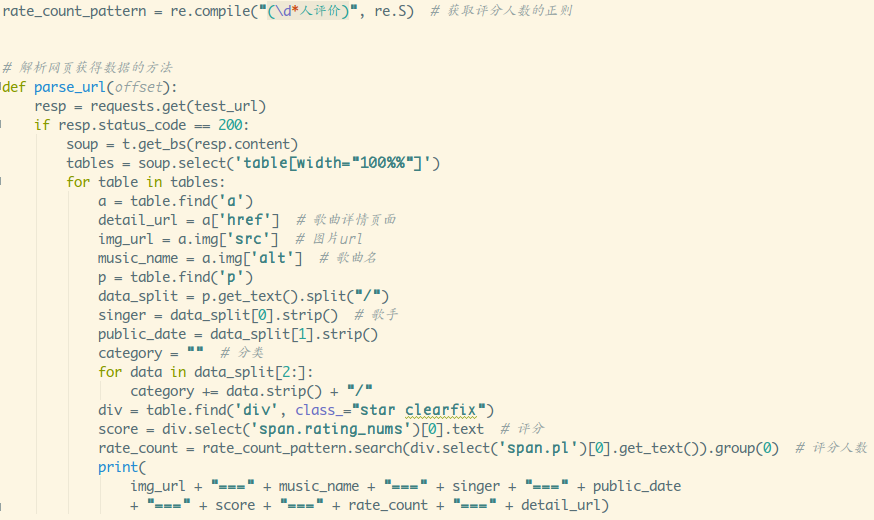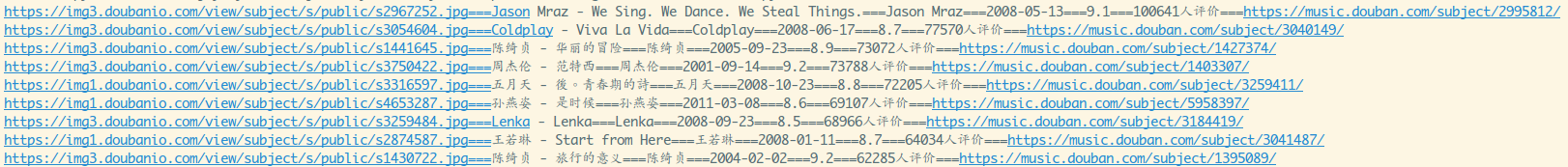## 2.如何将数据写入到Excel中

Step 1：安装库，操作Excel，你需要两个库：xlwt(写Excel) 和 xlrd(读Excel)

sudo pip3 install xlwtsudo pip3 install xlrd

Step 2熟悉几个基本函数

• xlwt.Workbook()：创建一个工作薄
• 工作薄对象.add_sheet(cell_overwrite_ok=True)：添加工作表，括号里是可选
参数，用于确认同一个cell单元是否可以重设值
• 工作表对象.write(行号，列号，插入数据，风格)，第四个参数可选
举个简单例子：插入这样的数据：
sheet.write(0,0,"姓名")sheet.write(0,1,"学号")sheet.write(1,0,"小猪")sheet.write(1,1,"No1")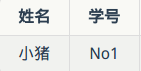• 工作薄对象.save(Excel文件名)：保存到Excel文件中

• xlrd.open_workbook()：读取一个Excel文件获得一个工作薄对象
• 工作薄对象.sheets()：根据索引获得工作薄里的一个工作表
• 工作表对象.nrows：获得行数
• 工作表对象.ncols：获得列数
• 工作表对象.row_values(pos)：读取某一行的数据，返回结果是列表类型的

## 3.编写一个Excel协助类

style：根据传入的字体名称，高度，是否加粗，返回一个Style样式
__init__：完成Excel表的一些初始化操作，初始化表头
insert_data：把爬取到的数据插入到Excel里的方法
read_data：读取Excel里数据的方法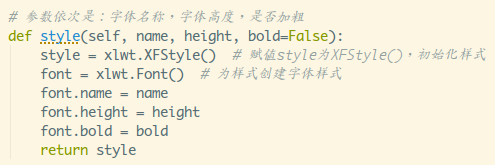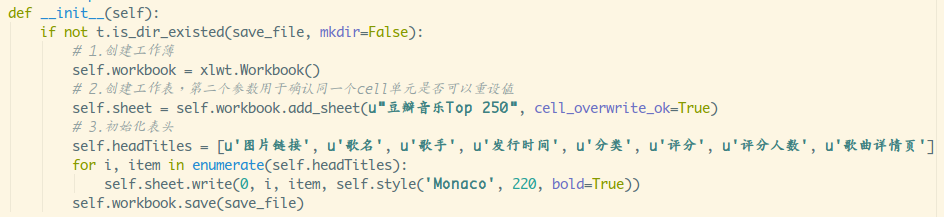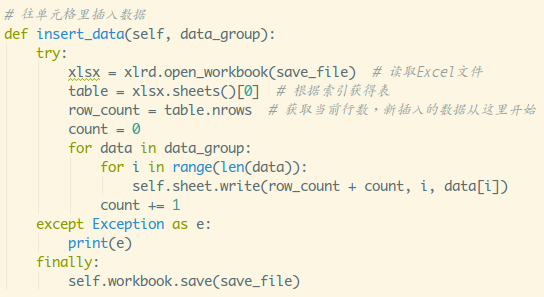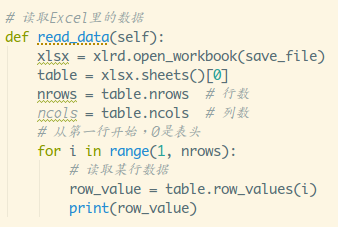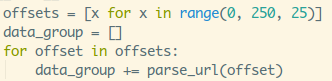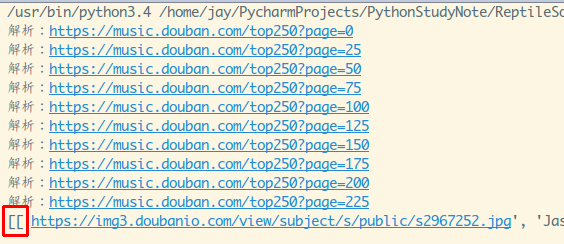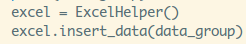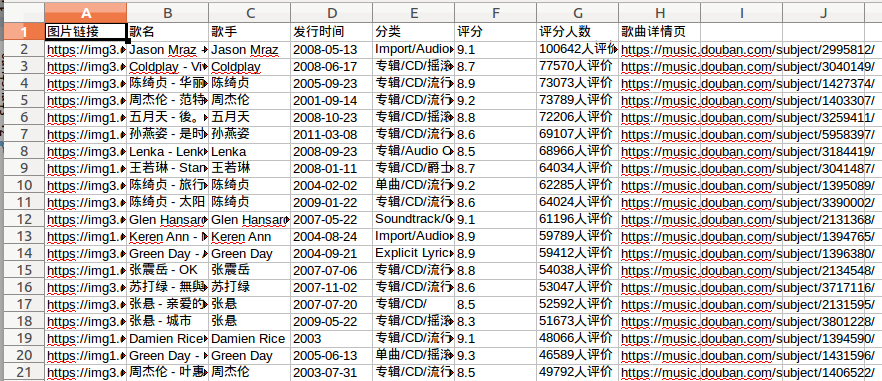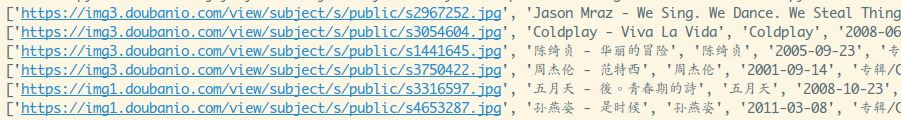## 4.小结

split()好多了，而且非开发者也能直接看懂，除此之外，哪天说不定可以撩到import reimport requestsimport xlwtimport xlrdimport tools as trate_count_pattern = re.compile("(\d*人评价)", re.S)  # 获取评分人数的正则base_url = 'https://music.douban.com/top250'save_file = 'dbyy.xlsx'# 解析网页获得数据的方法def parse_url(offset):    resp = requests.get(base_url, params={'page': offset})    print("解析：" + resp.url)    result = []    if resp.status_code == 200:        soup = t.get_bs(resp.content)        tables = soup.select('table[width="100%%"]')        for table in tables:            a = table.find('a')            detail_url = a['href']  # 歌曲详情页面            img_url = a.img['src']  # 图片url            music_name = a.img['alt']  # 歌曲名            p = table.find('p')            data_split = p.get_text().split("/")            singer = data_split.strip()  # 歌手            public_date = data_split.strip()            category = ""  # 分类            for data in data_split[2:]:                category += data.strip() + "/"            div = table.find('div', class_="star clearfix")            score = div.select('span.rating_nums').text  # 评分            rate_count = rate_count_pattern.search(div.select('span.pl').get_text()).group(0)  # 评分人数            result.append([img_url, music_name, singer, public_date, category, score, rate_count, detail_url])    return resultclass ExcelHelper:    def __init__(self):        if not t.is_dir_existed(save_file, mkdir=False):            # 1.创建工作薄            self.workbook = xlwt.Workbook()            # 2.创建工作表，第二个参数用于确认同一个cell单元是否可以重设值            self.sheet = self.workbook.add_sheet(u"豆瓣音乐Top 250", cell_overwrite_ok=True)            # 3.初始化表头            self.headTitles = [u'图片链接', u'歌名', u'歌手', u'发行时间', u'分类', u'评分', u'评分人数', u'歌曲详情页']            for i, item in enumerate(self.headTitles):                self.sheet.write(0, i, item, self.style('Monaco', 220, bold=True))            self.workbook.save(save_file)    # 参数依次是：字体名称，字体高度，是否加粗    def style(self, name, height, bold=False):        style = xlwt.XFStyle()  # 赋值style为XFStyle()，初始化样式        font = xlwt.Font()  # 为样式创建字体样式        font.name = name        font.height = height        font.bold = bold        return style    # 往单元格里插入数据    def insert_data(self, data_group):        try:            xlsx = xlrd.open_workbook(save_file)  # 读取Excel文件            table = xlsx.sheets()  # 根据索引获得表            row_count = table.nrows  # 获取当前行数，新插入的数据从这里开始            count = 0            for data in data_group:                for i in range(len(data)):                    self.sheet.write(row_count + count, i, data[i])                count += 1        except Exception as e:            print(e)        finally:            self.workbook.save(save_file)    # 读取Excel里的数据    def read_data(self):        xlsx = xlrd.open_workbook(save_file)        table = xlsx.sheets()        nrows = table.nrows  # 行数        ncols = table.ncols  # 列数        # 从第一行开始，0是表头        for i in range(1, nrows):            # 读取某行数据            row_value = table.row_values(i)            print(row_value)if __name__ == '__main__':    offsets = [x for x in range(0, 250, 25)]    data_group = []    for offset in offsets:        data_group += parse_url(offset)    print(data_group)    excel = ExcelHelper()    excel.insert_data(data_group)    excel.read_data()

PythonpythonpyPy加群交易屁眼 中的一个关键词即可通过；• 私有
• 公开
• 删除